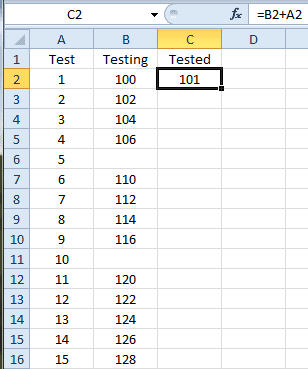# Fill Down a Formula with VBA

I commented on a post that brought to light, the fact that, using the cell fill-handle to “shoot” a formula down a column doesn’t always work when the adjacent column(s) have blank cells. So I decided to share some Excel VBA code that’s used to copy a formula down to the bottom of a column of data.

The situation is depicted below. Cell C2 is active, and has the formula =B2+A2. I want to copy it down to the rest of the column in this data range. However, cells B6 and B11 are empty, along with countless others below the visible table range. (Pretend this data table is huge.)Here is some VBA code that will Fill Down the formula.

``` Sub FillDownFormula() ''''''''''''''''''''''''''''''''''''''''''''''''''''''''''''' ' Filldown a formula for in column of data. ' Assumes a data table with headings in the first row, ' the formula in the second row and is the active cell. ''''''''''''''''''''''''''''''''''''''''''''''''''''''''''''' Dim rng As Range Dim rngData As Range Dim rngFormula As Range Dim rowData As Long Dim colData As Long```

``` ' Set the ranges Set rng = ActiveCell Set rngData = rng.CurrentRegion freestar.queue.push(function () { googletag.display('excelsemipro_300x600_300x250_160x600_unit_2'); }); ' Set the row and column variables rowData = rngData.CurrentRegion.Rows.Count colData = rng.Column ```

```' Set the formula range and fill down the formula Set rngFormula = rngData.Offset(1, colData - 1).Resize(rowData - 1, 1) rngFormula.FillDown End Sub ```

The key is setting the formula range (rngFormula). I take the entire region of data (rngData) and offset by 1 row because I don’t want the header row, then I resize the rows in the range by subtracting 1 from the total number of rows because I now need one less row in the range.

Next I offset the entire range by the row number of the active cell, but have to subtract one column because I offset from column 1, not column 0. And finally I resize the data range to 1 column, which gives me the single-column range I want with the formula in the top row.

The routine does no error checking and is restricted to using the active cell that has a formula. But it does the trick given those limitations.## 2 thoughts on “Fill Down a Formula with VBA”

1.Dennis Taylor

Things work differently in Excel 2010 for Windows (but not in Excel 2011 for the Mac).
When you double-click to copy cell content in Excel 2010, Excel looks to see if the data in adjacent columns to the left extend downward beyond the empty cell in the adjacent column, and if so, copies cell contents downward past the empty cells to the bottom of the data in the adjacent cells.

But if you are still working with Excel on the MAC or Excel 2007 and before, another workaround is to hide the adjacent column(s) to the left that have empty cells in them. Double-clicking the fill handle works off the first visible column to the left.

2.Gregory

I didn’t know you could hide an adjacent column like that. Nice tip.